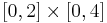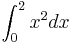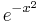# Exercises

## Introduction

Some exercises to get used to numpy

1. Make a vector of length 10 with elements all zero! Then modify its 4th element to 1 (zeros)
2. Make a 3-by-3 matrix with elements ranging from 0 up to 8 (reshape)
3. Make a random vector of length 30 containing random number between 0 to 1! Calculate its average and standard deviation! (rand, mean, std)
1. Make a random vector of the same length with elements between -3 and 2!
4. Make a random unit vector in 5 dimensions! First make a random vector in 5 dimensions and then normalize it to unit length!

## Monte-Carlo

Generate 500000 random points in the rectangle$[0,2]\times[0,4]$. Count how many of the points (x,y) have the property that x2 > y. Use this to approximate the integral$\int_0^2x^2 d x$ Like in the end of the lecture.

## Sorting Lambda

Write a Python program to sort a list of tuples using Lambda. Original list of tuples:

[('English', 88), ('Science', 90), ('Maths', 97), ('Social sciences', 82)]


Sorting the List of Tuples:

[('Social sciences', 82), ('English', 88), ('Science', 90), ('Maths', 97)]


## Lambda Selection

Write a Python program to filter a list of integers using Lambda. Original list of integers:

[1, 2, 3, 4, 5, 6, 7, 8, 9, 10]


Even numbers from the said list:

[2, 4, 6, 8, 10]


Odd numbers from the said list:

[1, 3, 5, 7, 9]


## Map Triples

Write a Python program to triple all numbers of a given list of integers. Use Python map.

# Homework 9

Each problem counts for 3 points

## Numeric integral

Estimate the integral of$e^{-x^2}$ on the interval [ − 2,5] with the left Riemann sum!

## Numeric derivative

Plot the function sin(x) and its derivative on the interval [ − π,π]. Calculate the derivative with finite difference method!